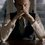# Freshman's Dream!

$\displaystyle \int_0^1 {x^{x^{x^{.^{.^.}}}} \ dx} = \sum_{k=0}^{\infty}{{(-1)}^k {(k+1)}^{k-1}}$

Proof:

Since generalized exponential series -

$\displaystyle \mathcal{E}_t(x) = \sum_{k = 0}^{\infty}{{(tk+1)}^{k-1} \frac{x^k}{k!}}$

follow

$\displaystyle \mathcal{E}_t(x) = \exp(x\mathcal{E}_t(x)^t)$

Therefore, for $t= 1$,

$\displaystyle \mathcal{E}(x) = \exp(x\mathcal{E}(x))$

$\displaystyle \mathcal{E}(\ln(x)) = \exp(\mathcal{E}(\ln(x)) \ln(x)) = x^{\mathcal{E}(\ln(x))}$

That's our tetration.

$\displaystyle \mathcal{E}(\ln(x)) = x^{x^{x^{.^{.^.}}}}$

Also,

$\displaystyle \mathcal{E}(\ln(x)) = \sum_{k = 0}^{\infty}{{(k+1)}^{k-1} \frac{{\ln(x)}^k}{k!}}$

$\displaystyle \int_0^1{x^{x^{x^{.^{.^.}}}}\ dx} = \sum_{k = 0}^{\infty}{\frac{{(k+1)}^{k-1}}{k!} \int_0^1{{(\ln(x))}^k \ dx} }$

Let's take this integral separately.

$I = \int_0^1 {{(\ln(x)}^k\ dx}$

Consider

$I(n) = \int_0^1 {x^n \ dx} = \frac{1}{n+1}$

$I^{(k)}(0) = \int_0^1 {{(\ln(x)}^k\ dx} = {(-1)}^k k!$

Therefore,

$\displaystyle \int_0^1{x^{x^{x^{.^{.^.}}}}\ dx} = \sum_{k = 0}^{\infty}{\frac{{(k+1)}^{k-1}}{k!}{(-1)}^k k! }$

$\displaystyle \int_0^1 {x^{x^{x^{.^{.^.}}}} \ dx} = \sum_{k=0}^{\infty}{{(-1)}^k {(k+1)}^{k-1}}$

/*

Now this "oscillating divergence" is really not what I liked. Is it correct, anyways?

I am not really freshman yet. Will be in a month or so.

*/Note by Kartik Sharma
4 years, 2 months ago

This discussion board is a place to discuss our Daily Challenges and the math and science related to those challenges. Explanations are more than just a solution — they should explain the steps and thinking strategies that you used to obtain the solution. Comments should further the discussion of math and science.

When posting on Brilliant:

• Use the emojis to react to an explanation, whether you're congratulating a job well done , or just really confused .
• Ask specific questions about the challenge or the steps in somebody's explanation. Well-posed questions can add a lot to the discussion, but posting "I don't understand!" doesn't help anyone.
• Try to contribute something new to the discussion, whether it is an extension, generalization or other idea related to the challenge.

MarkdownAppears as
*italics* or _italics_ italics
**bold** or __bold__ bold
- bulleted- list
• bulleted
• list
1. numbered2. list
1. numbered
2. list
Note: you must add a full line of space before and after lists for them to show up correctly
paragraph 1paragraph 2

paragraph 1

paragraph 2

[example link](https://brilliant.org)example link
> This is a quote
This is a quote
    # I indented these lines
# 4 spaces, and now they show
# up as a code block.

print "hello world"
# I indented these lines
# 4 spaces, and now they show
# up as a code block.

print "hello world"
MathAppears as
Remember to wrap math in $$ ... $$ or $ ... $ to ensure proper formatting.
2 \times 3 $2 \times 3$
2^{34} $2^{34}$
a_{i-1} $a_{i-1}$
\frac{2}{3} $\frac{2}{3}$
\sqrt{2} $\sqrt{2}$
\sum_{i=1}^3 $\sum_{i=1}^3$
\sin \theta $\sin \theta$
\boxed{123} $\boxed{123}$